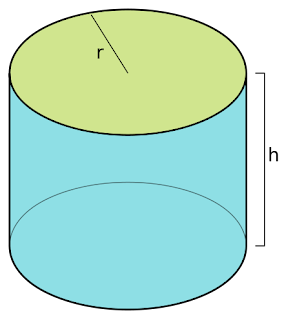## Cylinders Formulas for Competitive ExamsCylinder Definition: Cylinder is a 3d figure, in geometry, which has two parallel circular bases joined a curved surface at a particular distance from the centre. LPG gas-cylinder,  iron rods, plastic cold drinks cans are the real-life examples of cylinders.

Formulas:

Area of the circle of the Cylinder, A= πr²

Area of two circles of the cylinder, A= 2πr²

Curved Surface Area Cylinder, A =2πrh

Total surface area of the Cylinder, A = 2πr(r+h)

Volume of the Cylinder, V = πr²h

Cylinders Formulas for Competitive ExamsReviewed by SSC NOTES on February 13, 2023 Rating: 5